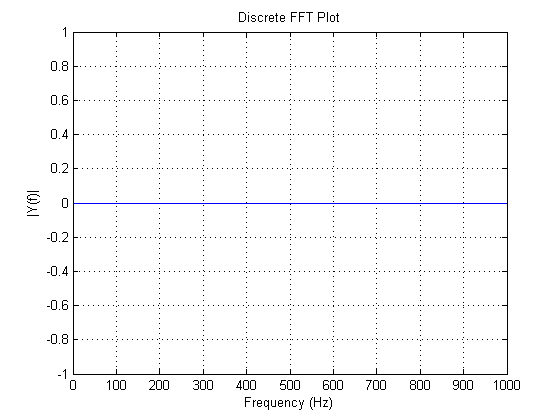# Acquire Continuous Audio Data

This example shows how to set up a continuous audio acquisition using a microphone.

### Create a DataAcquisition

Create a DataAcquisition with `directsound` as the vendor and add an audio input channel to it using `addinput`.

```dq = daq("directsound"); addinput(dq,"Audio0",1,"Audio"); ```

### Set Up the FFT Plot

```hf = figure; hp = plot(zeros(1000,1)); T = title('Discrete FFT Plot'); xlabel('Frequency (Hz)') ylabel('|Y(f)|') grid on; ```### Set ScansAvailableFcn

Update the figure with the FFT of the live input signal by setting the `ScansAvailableFcn`.

```dq.ScansAvailableFcn = @(src, evt) continuousFFT(src, hp); ```

### Start Acquisition

The figure updates, for 10 seconds, as the microphone is used.

```start(dq,"Duration",seconds(10)); figure(hf); ``````function continuousFFT(daqHandle, plotHandle) % Calculate FFT(data) and update plot with it. data = read(daqHandle, daqHandle.ScansAvailableFcnCount, "OutputFormat", "Matrix"); Fs = daqHandle.Rate; lengthOfData = length(data); % next closest power of 2 to the length nextPowerOfTwo = 2 ^ nextpow2(lengthOfData); plotScaleFactor = 4; % plot is symmetric about n/2 plotRange = nextPowerOfTwo / 2; plotRange = floor(plotRange / plotScaleFactor); yDFT = fft(data, nextPowerOfTwo); h = yDFT(1:plotRange); abs_h = abs(h); % Frequency range freqRange = (0:nextPowerOfTwo-1) * (Fs / nextPowerOfTwo); % Only plot up to n/2 (as other half is the mirror image) gfreq = freqRange(1:plotRange); % Update the plot set(plotHandle, 'ydata', abs_h, 'xdata', gfreq); drawnow end ```